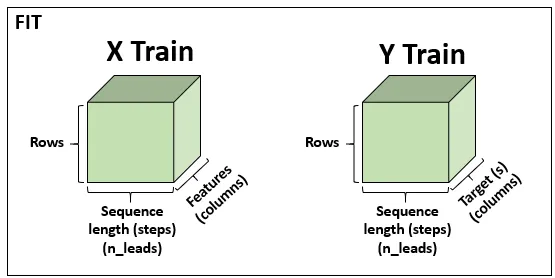# Regarding BiLSTM for timeseries and input/output tensors

Hello, I have a question regarding BiLSTM:Should both input and output tensors in the following format?I’m unsure how to interpret the visualizations you have posted, but maybe this excellent post or this one by @vdw could be helpful.

I assume you’re looking at a sequence labeling task like Named Entity Recognition or a Language Model. Basically, you use the output of each time step.

In this case, yes, in the input tensor and the output tensor will/should have those shapes. The only thing you have to be careful about is that you use a bidirectional LSTM. This means you have 2 sequence information.

I think I’ve got it. I am making a test with SantaFe Laser dataset and it looks fine. Bidirectional gets better precision:

Sorry but I still have some doubts regarding stacking BiLSTM layers.
I know this is done from nn.LSTM, but I would like to understand more in the process. I have tried to look at the code without success. Consider the following:

and suppose to have an Univariate timeseries dataset (I am not using for text).
The size of the input will be (I am considering batch_first=True):

(batch, seq, 1)

but the size of the output will be:

(batch, seq, 2 * hidden_layers)

now I suppose the input of the next layer should be again:

(batch, seq, 1)

So what is taken from the previous layer output? The last hidden layer?
According to what you wrote @ptrblck , could I do something like the following?

``````x = torch.randn(6, 4, 1)

rnn1 = nn.LSTM(1, 4, 10, bidirectional=True, batch_first=True)
rnn2 = nn.LSTM(1, 4, 10, bidirectional=True, batch_first=True)
h0 = torch.zeros(1, 4, 20)
c0 = torch.zeros(1, 4, 20)

output, (h_n, c_n) = rnn1(x, (h0, c0))

# Seperate directions
output = output.view(6, 4, 2, 10) #batch, seq_len, num_directions, hidden_size

# Last hidden layer output
new_input = output[:, :, 1, -1].unsqueeze(-1)

# second layer
output, (h_n, c_n) = rnn2(new_input, (h0, c0))
# or ?
output, (h_n, c_n) = rnn2(new_input, (h_n, c_n))

``````

This part seems off to me

Firstly, what do you mean by “last layer” here? `output` will only contain the last layer of you defined your `nn.LSTM` with `num_layers` greater than 1.

Also `output[:, :, 1, -1]` gives you the last values of the hidden dimensions for the backward pass. Why would you do that?

My recommendation:

``````output = output.view(6, 4, 2, 10) #batch, seq_len, num_directions, hidden_size

fwd = output[:, :, 0, :]
bwd = output[:, :, 1, :]
new_input = fwd + bwd
``````

The shape should be `(batch_size, seq_len, hidden_size)`. This means that you have to define a 2nd RNN layer that expects in your example now 10 as input size. I don’t think you should simply throw away 9 of your 10 values of the hidden dimensions just so it fits as input for `rnn`.

Why don’t you just use `nn.LSTM` with `num_layers=2`? It handles all this for you under the hood.

The reason I am asking it is because I am trying to reproduce the results of this paper:

For this reason at each layer I need to concatenate some additional features:Now consider each LSTM layer, it is a transformer:

LSTM: (batch, seq_len, 1) → (batch, seq_len, 1)

and in fact the additional input I need to add to each layer has dimension (batch, seq_len, 1).
Honestly I can’t understand how this concatenation need to be done. I have tried to concatenate the full output with the X features, but I am not convinced and I am not getting good results.

In particular this document says:

The linear observation is treated as the additional input vector for each layer of the stacked BiLSTM layers for prediction and the nonlinear observation is connected to the bottom of the multiple BiLSTM layers. Meanwhile the output of the previous BiLSTM layer is connected to the linear observation vector to form a new input signal for the next BiLSTM layer.

and:

and:

So I wonder to know if the output is expected to be of the same dimensions of the target variable or it has the hidden_dimension as 3D shape? Because the fully connected layer is supposed to be applied only at the end.

It is not so bad the solution you gave me @vdw .
It is very good with Solar Santa Fe dataset:

and quit good with sunspot dataset:

I am checking if any improvement can be done.

It seems there was a data leak. I am not happy, it does not learn: